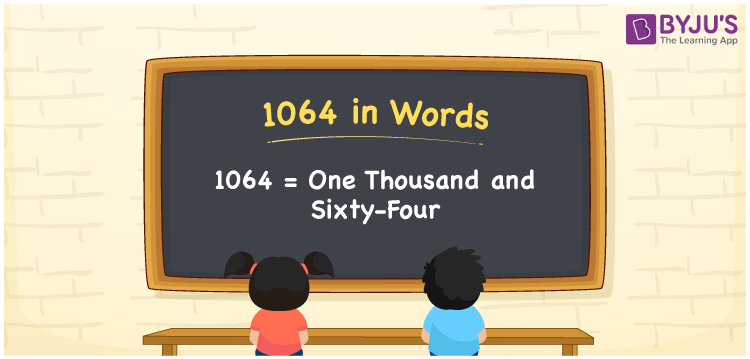# 1064 in words

1064 in words is written as One Thousand and Sixty Four. 1064 represents the count or value. The article on Counting Numbers can give you an idea about count or counting. The number 1064 is used in expressions that relate to money, distance, length, year and others. Let us consider an example for 1064. ”The year One Thousand and Sixty Four was a leap year”.

 1064 in words One Thousand and Sixty Four One Thousand and Sixty Four in Numbers 1064

## 1064 in English Words## How to Write 1064 in Words?

We can convert 1064 to words using a place value chart. The number 1064 has 4 digits, so let’s make a chart that shows the place value up to 4 digits.

 Thousands Hundreds Tens Ones 1 0 6 4

Thus, we can write the expanded form as:

1 × Thousand + 0 × Hundred + 6 × Ten + 4 × One

= 1 × 1000 + 0 × 100 + 6 × 10 + 4 × 1

= 1064

= One Thousand and Sixty Four.

1064 is the natural number that is succeeded by 1063 and preceded by 1065.

1064 in words – One Thousand Sixty Four.

Is 1064 an odd number? – No.

Is 1064 an even number? – Yes.

Is 1064 a perfect square number? – No.

Is 1064 a perfect cube number? – No.

Is 1064 a prime number? – No.

Is 1064 a composite number? – Yes.

## Solved Example

1. Write the number 1064 in expanded form

Solution: 1 × 1000 + 0 × 100 + 6 × 10 + 4 × 1

We can write 1064 = 1000 +000 + 60 + 4

= 1 × 1000 + 0 × 100 + 6 × 10 + 4 × 1

## Frequently Asked Questions on 1064 in words

Q1

### How to write 1064 in words?

1064 in words is written as One Thousand and Sixty Four.
Q2

### Is 1064 a perfect square number?

No. 1064 is a not perfect square number.
Q3

### Is 1064 a prime number?

No. 1064 is not a prime number.